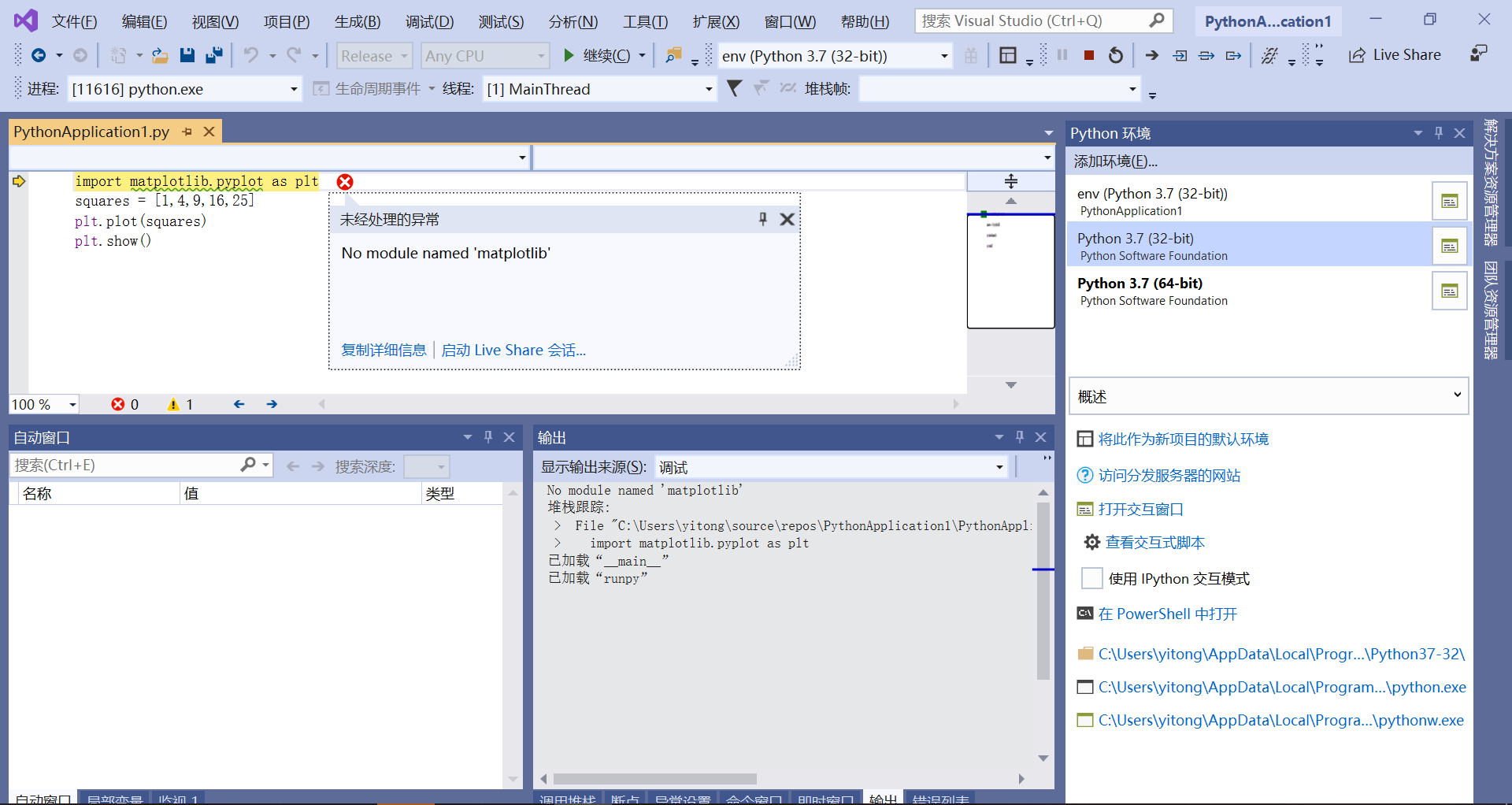vs中使用python操作matplotlib时发生错误1个回答python3.8下载matplotlib模块时，总是出现以下错误 ERROR: Command errored out with exit status 1: 'c:\users\air\python\python38\python.exe' -u -c 'import sys, setuptools, tokenize; sys.argv = '"'"'C:\\Users\\air\\AppData\\Local\\Temp\\pip-install-5ntug3if\\matplotlib\\setup.py'"'"'; __file__='"'"'C:\\Users\\air\\AppData\\Local\\Temp\\pip-install-5ntug3if\\matplotlib\\setup.py'"'"';f=getattr(tokenize, '"'"'open'"'"', open)(__file__);code=f.read().replace('"'"'\r\n'"'"', '"'"'\n'"'"');f.close();exec(compile(code, __file__, '"'"'exec'"'"'))' install --record 'C:\Users\air\AppData\Local\Temp\pip-record-rp63qqyg\install-record.txt' --single-version-externally-managed --compile Check the logs for full command output. ![图片说明](https://img-ask.csdn.net/upload/201911/20/1574238491_785213.png) 有那位大神可以帮我看一下？

python用matplotlib画K线

import matplotlib.pyplot as plt import matplotlib.finance as mpf with open('SH#600004.txt') as obj: text=obj.readlines() baseinfo=text dayinfo=text[2:-1] date_list =[dayinfo[i].split(',') for i in range(len(dayinfo))] open_list =[dayinfo[i].split(',') for i in range(len(dayinfo))] high_list =[dayinfo[i].split(',') for i in range(len(dayinfo))] low_list =[dayinfo[i].split(',') for i in range(len(dayinfo))] close_list=[dayinfo[i].split(',') for i in range(len(dayinfo))] quotes=zip(date_list,open_list,high_list,low_list,close_list) #N=100 #open_list[:N],high_list[:N],low_list[:N],close_list[:N] fig,ax=plt.subplots() mpf.candlestick_ohlc(ax,quotes,width=0.6,colorup='r',colordown='green') plt.title(baseinfo) plt.xlabel(date_list) plt.rcParams['font.sans-serif']=['SimHei'] #用来正常显示中文标签 plt.rcParams['axes.unicode_minus']=False #用来正常显示负号 plt.show() **#以下是报错信息 C:\ProgramData\Anaconda3\python.exe G:/python/test/test.py C:\ProgramData\Anaconda3\lib\site-packages\matplotlib\cbook\deprecation.py:106: MatplotlibDeprecationWarning: The finance module has been deprecated in mpl 2.0 and will be removed in mpl 2.2. Please use the module mpl_finance instead. warnings.warn(message, mplDeprecation, stacklevel=1) Traceback (most recent call last): File "G:/python/test/test.py", line 18, in <module> mpf.candlestick_ohlc(ax,quotes,width=0.6,colorup='r',colordown='green') File "C:\ProgramData\Anaconda3\lib\site-packages\matplotlib\finance.py", line 737, in candlestick_ohlc alpha=alpha, ochl=False) File "C:\ProgramData\Anaconda3\lib\site-packages\matplotlib\finance.py", line 794, in _candlestick height = open - close TypeError: unsupported operand type(s) for -: 'str' and 'str'** 我搜了下知道日期格式转换需要用到date2num这个函数 我的文本里面日期格式是XXXX/XX/XX的形式 请问quotes一项里日期需求的格式是什么?

Python使用matplotlib绘图怎么把图的标题放到图下方

 plt.title('助攻表现（常规赛）') plt.ylabel('场均助攻数') plt.xlabel('年份-冠军球队') plt.ylim(17) plt.setp(plt.xticks(idx,tick),rotation=90) plt.legend(bbox_to_anchor=(1.15,1)) plt.show()  怎么修改呢，像R那样把标题放在图下面

python安装matplotlib报错

python用matplotlib显示图片出错

python用matplotlib画图时如何使坐标轴的数字显示为千分位的形式如100,000,000

python用matplotlib画图时如何使坐标轴的数字显示为千分位的形式如100,000,000

python import matplotlib.pyplot 报错 DLL load failed

Anaconda3.5 python3.6 pycharm win7系统 import matplotlib没问题 matplotlib 2.1.0 import matplotlib.pyplot 报错 ![图片说明](https://img-ask.csdn.net/upload/201712/07/1512653783_182335.png) 另外，import matplotlib之前有个错误，更新了numpy之后好了。 在网上没找到类似的问题

Python/matplotlib：已知空间上一点坐标和长宽高值，如何画出立方体？

python的matplotlib中根据分类选取不同标记

python matplotlib.pyplot画折线图

python matplotlib 画两条折线图 其中一组数据有空值 如何处理？

python matplotlib 画两条折线图 其中一组数据有空值 如何处理？ import csv from datetime import datetime from matplotlib import pyplot as plt filename = 'data.csv' with open(filename) as f: reader = csv.reader(f) header_row = next(reader) dates = [] temp1 = [] temp2 = [] for row in reader: time = row + '-' + row + '-' + row current_date = datetime.strptime(time, "%Y-%m-%d") dates.append(current_date) tempm1 = int(float(row)) temp1.append(tempm1) tempm2 = int(row) temp2.append(tempm2) fig = plt.figure(dpi=128, figsize=(10,6)) plt.plot(dates, temp1, c='red') plt.plot(dates, temp2, c='blue') plt.show() 报错： C:\Users\yo\AppData\Local\Programs\Python\Python37\python.exe F:/论文/data/compare/compare.py Traceback (most recent call last): File "F:/论文/data/compare/compare.py", line 26, in <module> tempm2 = int(row) ValueError: invalid literal for int() with base 10: '20.075' Process finished with exit code 1 试过if 不为空值处理，然后数据对不齐了 我想要如果是空值图上就不显示 数据是有小数的所以就用int float 处理 请各位大神帮忙 谢谢！

ubuntu16.04 python2.7环境下使用matplotlib不能画图

Python中matplotlib.pyplot.hist显示x must be 1D or 2D

“亚马逊丛林里的蝴蝶扇动几下翅膀就可能引起两周后美国德州的一次飓风……” 这句人人皆知的话最初用来描述非线性系统中微小参数的变化所引起的系统极大变化。 而在更长的时间尺度内，我们所生活的这个世界就是这样一个异常复杂的非线性系统…… 水泥、穹顶、透视——关于时间与技艺的蝴蝶效应 公元前3000年，古埃及人将尼罗河中挖出的泥浆与纳特龙盐湖中的矿物盐混合，再掺入煅烧石灰石制成的石灰，由此得来了人...

loonggg读完需要3分钟速读仅需 1 分钟大家好，我是你们的校长。我之前讲过，这年头，只要肯动脑，肯行动，程序员凭借自己的技术，赚钱的方式还是有很多种的。仅仅靠在公司出卖自己的劳动时...

MySQL数据库面试题（2020最新版）

HashMap底层实现原理，红黑树，B+树，B树的结构原理 Spring的AOP和IOC是什么？它们常见的使用场景有哪些？Spring事务，事务的属性，传播行为，数据库隔离级别 Spring和SpringMVC，MyBatis以及SpringBoot的注解分别有哪些？SpringMVC的工作原理，SpringBoot框架的优点，MyBatis框架的优点 SpringCould组件有哪些，他们...

《经典算法案例》01-08：如何使用质数设计扫雷（Minesweeper）游戏

《Oracle Java SE编程自学与面试指南》最佳学习路线图（2020最新版）